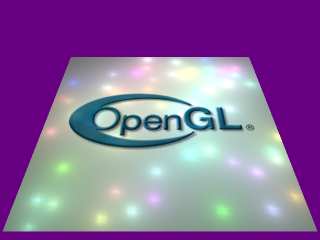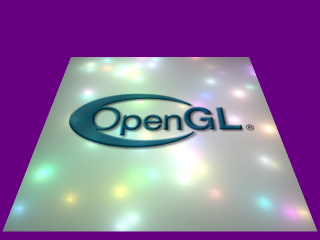# Many Lights## Description:

This simple demo displays a well-tesselated quad, with 60 point lights moving above it. Three methods of lighting the quad are used.

#### Method 1

Using the fixed function pipeline eight passes are required, each using the eight standard OpenGL lights.

#### Method 2

A vertex program (NV_vertex_program) can be enabled when the quad is drawn. This calculates the vector from the vertex to the current light, normalises it and dots it with the normal to the quad. This is then modulated by the light's color and added to an accumulator. Thus 6 instructions are required for each light, so 20 lights can be considered in a single pass. Using NV_vertex_program, the 60 lights are calculated in 3 passes using additive blending.

#### Method 3

NV_vertex_program was chosen instead of the ARB version because this program is my first use of the NV_vertex_program2 extension which allows looping and function calls in a vertex program. The NV_vertex_program2 program has a subroutine to calculate the lighting contribution for a single light. The main part of the vertex program then uses a simple loop to calculate all 60 lights in one pass.

## Requirements:

No Extensions required.

#### Recommended:

NV_vertex_program
NV_vertex_program2

## References:

Extension specifications for the above.